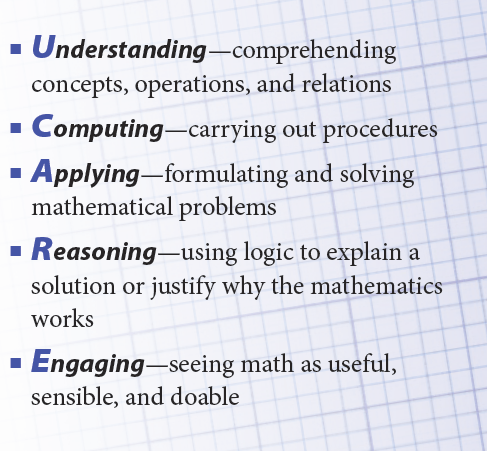## What Is Math-Curriculum 2.0?

### Deep Understanding

The Curriculum 2.0 (C2.0) Mathematics program develops a deep understanding of mathematics by building a strong foundation of number sense at the elementary level before moving into more advanced content.

Students who have a deep understanding of mathematics content

• make sense of problems and persevere in solving them
• reason abstractly and quantitatively
• construct viable arguments and critique the reasoning of others
• represent real-world situations with mathematical modeling
• use appropriate tools strategically (manipulatives, calculators, etc.)
• use definitions, calculations, and estimations with the appropriate level of precision
• look for and make use of patterns and structure
• and look for and express generalities within mathematics.

### Proficiency

Students who deeply understand a mathematical concept are referred to as proficient in that concept. Proficiency is defined in the five intertwined strands of UCARE.